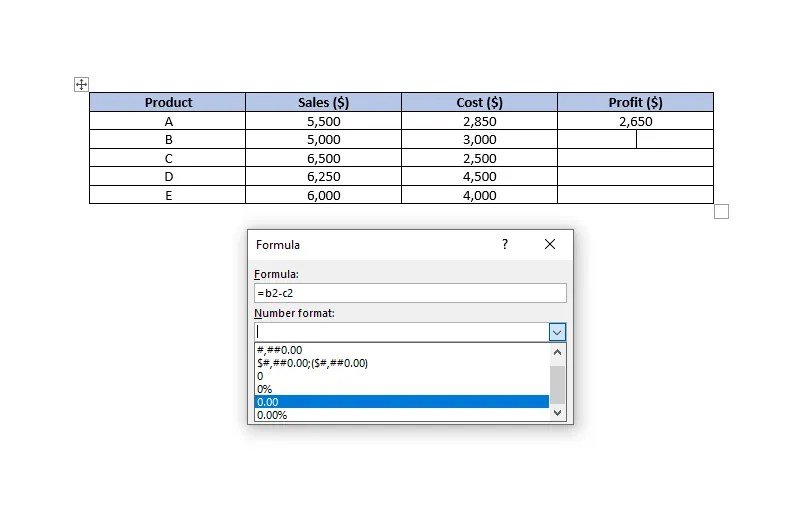Cloudorian Technology
Take a fresh look at Tech.

# The Best Way to Incorporate Excel Formulas Into Ms Word documents

## How to Use Excel Formulas in Word Documents

0 398

Get real time updates directly on you device, subscribe now.

Adding an entire Excel workbook to a Word page is overkill when all you need is a simple table. A table and Excel formulas can be easily integrated into a Word document, thanks to the ease with which they can be created. However, the number of formulas available is restricted.

It’s possible to put sales data into a table by adding columns for sales, total cost, and profit calculation using formulas, all of which might be combined into one table. You can also compute an average or a maximum for each of these columns.

## Method 1: Copy and Paste Data from a Spreadsheet Into a Word Document

You May Also Like:

1. Copy the cells containing the data and open a Word document.
2. From the top ribbon, click on the arrow under the Paste button, and click on Paste Special.Image Credit: MakeUseOf
3. You’ll see a new window pop-up where you’ll need to select what you want to paste the copied content as. Select Microsoft Excel Worksheet Object and select OK.Image Credit: MakeUseOf
4. Your data should now appear in the Word document, and the cells should contain the formulas as well.

If you need to make any changes, simply double-click on the pasted information to turn your Word document into an Excel spreadsheet. From there, you may make any changes you need.

## Method 2: Using Word’s Table Cell Formulas

1. Quickly insert a table in your Word document and populate the table with data.
2. Navigate to the cell where you want to make your computations using a formula. Once you’ve selected the cell, switch to the Layout tab from the ribbon at the top and select Formula from the Data group.

Notice that there are two tabs called Layout. You need to select the one that appears under Table Tools in the ribbon.

3. When you click on Formula, you’ll see a small window pop up.
4. The formula you want to use goes in the first field of the text box. You may conduct simple arithmetic operations as well as formulas here. For example, if you want to figure out how much profit you’ll make, you can use the formula.:
`=B2-C2`

Here, B2 represents the second cell in the second column, and C2 represents the second cell in the third column.

5. The Number Format can be customized in the second field. Choose a number format in such a way that your profit may be calculated down to the second decimal place, for example.Image Credit: MakeUseOf

6. The Paste Function field lists the formulas you can use in Word. If you can’t remember the name of a function, you could select one from the dropdown list, and it will automatically be added to the Formula field.
7. When you’ve entered the function, click OK, and you’ll see the computed figure in the cell.

## Positional Arguments

Positional arguments (ABOVE, BELOW, LEFT, RIGHT) can often make things simpler, especially if your table is relatively large. For instance, if you have 20 or more columns in your table, you could use the formula =SUM(ABOVE) instead of referencing each cell inside the parenthesis.

Positional arguments can be used with the subsequent functions:

• SUM
• AVERAGE
• MIN
• MAX
• COUNT
• PRODUCT

For example, we might use the formula to figure out what the average sales are in the previous case:

`=AVERAGE(ABOVE)`

You can combine positional arguments if your cell is in the middle of the column. For example, you could use the formula below to add up the values in a specific cell’s range.:

`=SUM(ABOVE,BELOW)`

You can use the following formula in a corner cell to add the values from both the row and column:

`=SUM(LEFT,ABOVE)`

The few capabilities that Word provides are fairly powerful and will easily allow you to generate the majority of tables without any issues with functioning.

## Updating Data and Findings

Word, in contrast to Excel, does not automatically update formula results as they change. It does, however, update the results upon closing and reopening the document once it has been processed. Keep things simple by only making updates to the data, then saving and reopening the document.

As long as the document is open, you can update the formula results by selecting the formula results (and not just the cell) and right-clicking on them. Then choose Update Field.

As soon as you click on Update Field, the formula’s output should be updated right away.

## Cell References

In Word, you may refer to a cell in a number of different ways.

### 1. Bookmarknames

Assume you’ve named your average sales value “average_sales” in your bookmarks. When in doubt about how to name a cell as a bookmark, go to the ribbon at the top, pick the cell, and then click on Insert > Bookmark.

You want to convert a decimal figure to an integer, therefore let’s assume the average sales value is a decimal. The average sales amount can be referenced as ROUND(average sales,0), which will reduce the number to the closest integer.

### 2. RnCn References

You can use the RnCn referencing convention to refer to a table’s row, column, or a particular cell. There are nth rows in this table, and nth columns in this table are represented by Rn and Cn, respectively. For example, R2C5 would be used to refer to the fifth column and second row.

You can even select a range of cells using the RnCn reference, much like you would in Excel. For instance, selecting R1C1:R1C6 selects the first six cells of the first row. For selecting the entire row in which you’re using the formula, just use R (or C for a column).

Source MakeUseOf
You might also like